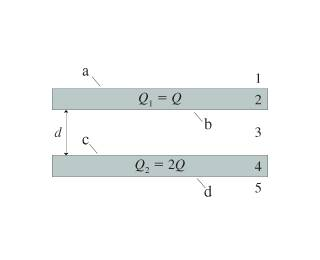# Electromagnetics field question

• Archived

## Homework StatementThe figure shows two very large slabs of metal that are parallel and distance l apart. Each slab has a total surface area (top + bottom) A. The thickness of each slab is so small in comparison to its lateral dimenstions that the surface area around the sides is negligible. Metal 1 has total charge = Q1 = Q. Assume Q is positive. In terms of Q and A, determine

a. The electric field strengths E1 to E5 in regions 1 to 5.
b. The surface charge densities na to nd on the four surfaces a to d.

2. The attempt at a solution

E1 = Q/E0
because the area vector is opposite to the bottom plate
E4 = E2 = 0 (in a conductor, E is 0)
E5 = Q/(2E0)
because the area vector is opposite to the top plate

I do not know how to determine E3, and I have no clue how to do part b